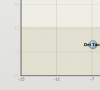## Recommended Posts

Hi there,

I am creating a bubble chart with fusionchart-xt and the xml format.  I have problems with the vertical scale.  I want the horizontal lines to have an opacity of 0.15 and the labels of 1.  I cannot succeed to do it.  In short, I want the vertical scale similar to the horizontal one in the attach image.

I attach an xml file and image.

cheers!

didier

bubble.xml##### Share on other sites

Hi,

As per your shared XML data, this JSFiddle is created : http://jsfiddle.net/vishalika/58sYu/377/

Can you check and point out the requirement in this?

Awaiting response.

##### Share on other sites

Thanks Vishalika,

I did not succeed to save the fiddle but you will find bellow the xml that generated the pale y axis.

cheers!

didier

```<graph exportEnabled="0"  showYAxisValues="0" divLineAlpha="0" showLimits="0" yAxisMinValue="-9.0" xAxisMinValue="-0.110731989318" showAboutMenuItem="0" yAxisMaxValue="32.0" showAlternateHGridColor="0" canvasPadding="0" showZeroPlane="0" showToolTip="1" showVLimits="0" xAxisMaxValue="0.582691758319" yAxisName="Value"  bubbleScale="0.7" subcaption="Latest data is from 14/09/2015">
<categories>
<category showVerticalLine="1" x="-0.110731989318" label="-0.1"/>
<category showVerticalLine="1" x="-0.010731989318" label="-0.0"/>
<category showVerticalLine="1" x="0.089268010682" label="0.1"/>
<category showVerticalLine="1" x="0.189268010682" label="0.2"/>
<category showVerticalLine="1" x="0.289268010682" label="0.3"/>
<category showVerticalLine="1" x="0.389268010682" label="0.4"/>
<category showVerticalLine="1" x="0.489268010682" label="0.5"/>
<category showVerticalLine="1" x="0.582691758319" label="0.6"/>
</categories>
<dataset showValues="1">
<set name="sdsdsd" color=""
x="0.3"
y="25.5"
z="28.1"
tooltext="dsssd: X.1"
/>
<set name="sdsdsd" color=""
x="0.3"
y="0.4"
z="5.0"
tooltext="0"
/>
<set name="sddssd" color="FF5555"
x="-0.0"
y="-2.5"
z="0.9"
tooltext="sddsds.9"
/>
<set name="sdjdsd" color=""
x="0.5"
y="3.7"
z="7.2"
tooltext="2"
/>
</dataset>
<trendlines>
<line alpha="15" color="948A54" displayValue=" " endValue="2.05934018754" isTrendZone="1" startValue="-9.0"/>
<line alpha="15" startValue="-9.0" displayValue="-9"/>
<line alpha="15" startValue="-3.0" displayValue="-3"/>
<line alpha="15" startValue="3.0" displayValue="3"/>
<line alpha="15" startValue="9.0" displayValue="9"/>
<line alpha="15" startValue="15.0" displayValue="15"/>
<line alpha="15" startValue="21.0" displayValue="21"/>
<line alpha="15" startValue="27.0" displayValue="27"/>
<line alpha="15" startValue="32.0" displayValue="32"/>
</trendlines>
<vtrendlines>
<line alpha="15" color="948A54" displayValue=" " endValue="0.310206573411" isTrendZone="1" startValue="-0.11"/>
</vtrendlines>
<styles>
<definition>
<style size="10" color="000000" bold="1" name="boldfont" type="font" />
<style size="10" color="555555" bold="1" name="subcaptionfont" type="font" />
<style size="10" color="000000" bold="0" name="defaultfont" type="font" />
</definition>
<application>
<apply toObject="CAPTION" styles="boldfont" />
<apply toObject="SUBCAPTION" styles="subcaptionfont" />
<apply toObject="XAXISNAME" styles="defaultfont" />
<apply toObject="YAXISNAME" styles="defaultfont" />
<apply toObject="DATAVALUES" styles="boldfont" />
</application>
</styles>
</graph>
```

Hi,

Hope this helps.

##### Share on other sites

Hi Vishalika,

Thanks a lot for your help.  On the fiddle you shared, all the axis are light, I need them darker.  Only the axis labeld ( -9, -3, 3 , ...) not the grid itself.  Can we do that with fusion charts?

thanks

didier

##### Share on other sites

Hi,

Try setting the alpha value in the category element of the JSFiddle shared.

Hope this helps.